by Chris Bentley

## Introduction

In computer graphics objects are often rendered without shadows, and appear not to be anchored in the environment. Shadows convey a large amount of information because they provide what is essentially a second view of an object. This presentation (web page) will discuss two popular algorithms for generating shadows when rendering polygon mesh models.

Object rendered with no shadow, appears to float above plane:A point is in shadow relative to a given light source if rays from that light source cannot directly reach the point. Stated another way: shadowed points are points that the light cannot "see". Point light sources produce shadows with "hard" edges. Non-point light sources produce both umbra and penumbra shadows. Here, I will only consider point light sources, although extending one of the shadow algorithms to handle area light sources would not be difficult.

Numerous methods exist to render shadows using polygonal mesh data:
1. Transforming polygons to "ground", creating shadow polygons for each object polygon
3. Calculating shadow pixels by tracing rays from points on object to light source location.
The discussion in this presentation will focus on algorithms 1 and 2, considering only the case of point light sources, which produce hard edges.

## I. Transforming Polygons to Ground

This method is descibed by Jim Blinn, [BLIN88]. In this article, Blinn describes the equations for transforming a polgyon onto the z = 0 plane, opposite the direction that the light is shining from. He discusses two cases:
1. light at infinity
2. local light source
This method uses the geometric relationship of light sources and polygons, i.e. similar triangles, to calculate each polygon's projection on z = 0 plane. The "shadow polygon" generated in this way should be generated for every light source. So for N lights there will be N projections of each polygon.

Case 1. light at infinity

Light source at infinity: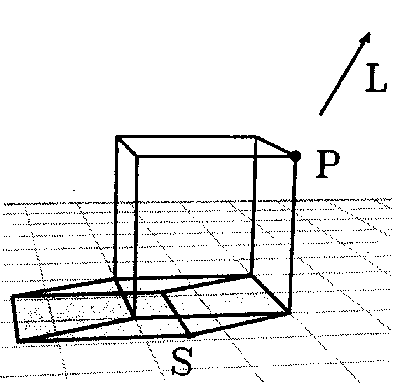For the case of a light source positioned infinitely far away, we will assume that all the rays reaching the object are parallel. This will allow us to solve the shadow equations once, and apply the solutions to every vertex in our object. Given 2 points:

1. light point,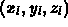2. vertex point,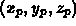We want to calculate:

`     `
shadow point,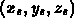From similar triangles we have:Solving for: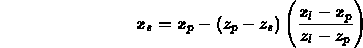If L is vector from point (P) to the light, then the Point-Vector form of the line is:Since we require that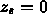, this becomes:orThen, solving for: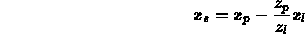withbeing similar.

In matrix form:Now given the world coordinates of any polygon vertex, P , we can multiply: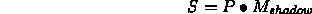This computes the projected shadow points of the polygon, which we can fill, producing a shadow polygon.

Shadows using "ground transformation" with two light sources at infinity:Case 2. local light sources

Perspective shadow from local light source: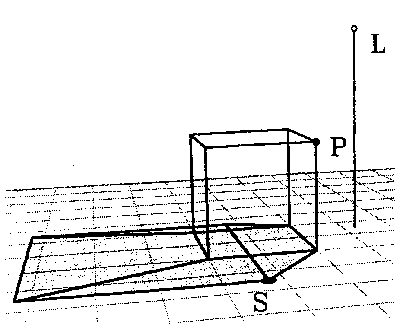The equations for an infinite light source with parallel rays can be extended for the case of light sources that are positioned at some point in space, a finite distance away from the object being rendered. Note that now we will need to perform an additional calculation for every vertex in our object, because each vertex will, in general, have a different vector to the light. However, in this case too we can place most of our calculations in a matrix.

If, now, l is the location of the light source, and (P) is the polygon vertex, we can again use the Point-Vector form of the line:Again, we require that, so:and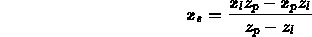withbeing similar.

By using the division performed when turning homogeneous coordinates into 3D coordinates, we can write the matrix: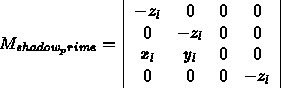Again, given the world coordinates of any polygon vertex, P , we can multiply:and then homogenize to compute projected shadow point.

Shadows using "ground transformation" with local light source: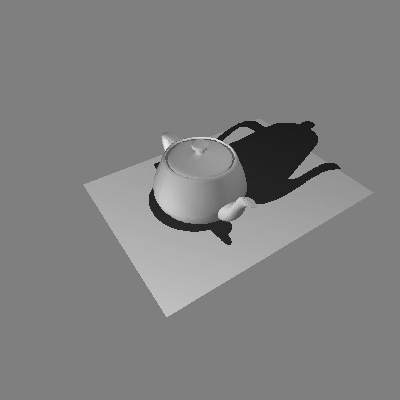## Implementation of Ground Transformation

Here is the code to load the shadow transformation matrix, W :
```	/*
* get world coordinates of light
*/
copy_vect( light_point, view->lights[n]->world_coords );

/*
*/
ident_mat( W );
W = -light_point;
W = light_point;
W = -light_point;
W = light_point;
W = 0;
W = -light_point;
```
And here is the code for multiplying a polygon's world coordinates by the shadow matrix to project the polygon onto the z = 0 plane:
```	/*
* transform object world coordinates into z = 0 plane, using W matrix
*/
pt_matrix_mult( wpt, W, v[i].world_coords );
homo( v[i].world_coords );

/*
* transform new coordinates of shadow point by viewing
* and perspective transformations
*/
pt_matrix_mult( v[i].world_coords,cur_view->VPN,v[i].screen_coords);
homo( v[i].screen_coords );
```

Adapting the Z-buffer hidden surface removal algorithm in order to calculate shadows was first described by Williams [WILL78]. This method follows directly from the idea that shadow points are "hidden" from light. In other words, shadows are "hidden surfaces" from the point of view of a light. If we pretend that the light point is the center of projection (i.e an eye point), we can render the scene from the light's point of view, using a Z-buffer to compute surfaces visible to the light. The Z-buffer resulting from this will record all of the points that are closest to the light. Any point that has a "farther" Z value at a given pixel is invisible to the light and hence is in shadow.

The Z-buffer method involves looking at the object from the point of view of each light in the scene, and computing a Z-buffer of the object as seen by each light. After this preprocessing is performed, the object is rendered from the "true" eye position. For every pixel visible to the eye, we will transform the object point into the light's view to determine whether that point was also visible to the light. If it was not, then that point is in shadow.

Note that when we are calculating the hidden surfaces from the point of view of each light source, we only care about the depth information, and we are not interested in performing lighting calculations for these polygons, because the "light's eye views" will not normally be seen by the user. This permits faster rendering when precalculating the shadow Z-buffers.

## Implementation of Shadow Z-buffer Algorithm

Precomputing phase

```1.0  for each light source
1.1      make light point be center of projection
1.2      calculate transformation matrices
1.3      transform object using light point matrices
1.4      render object using zbuffer - lighting is skipped
1.5      save computed zbuffer (depth info)
```

Object rendering phase

```2.0  make eye point be center of projection
3.0  recalculate transformation matrices
4.0  transform object using eye point matrices
5.0  render object using zbuffer

5.1      for every pixel visible from eye
5.1.1        transform world point corresponding to pixel to shadow coordinates
5.1.2        for every light source
5.1.2.1          sample saved zbuffer for that light
5.1.2.2          if shadow coordinates < zbuffer value
```

## Problems in Shadow Z-buffer Algorithm

The shadow Z-buffer algorithm has two serious problems relating to how the precomputed Z-buffers are sampled. To see the first problem consider a point that is visible to both the eye and a light. Assume the shadow Z-buffer for the light has already been computed. When transforming the point's world coordinates to shadow coordinates the point will (ideally) project in the shadow Z-buffer to the same spot that this point projected to when viewed from the light. If this is the case, though, the algorithm may decide that the point is in shadow. Also, due to inaccuracies in the projection calculations, the point may project to a spot in the shadow Z-buffer that has a slightly "nearer" z value. In other words, points can appear in shadow, because they shadow themselves, or because we are mistakenly comparing them with their neighbors!

The solution to the problem of points "shadowing themselves" is to cheat a little: when transforming the point into shadow coordinates to see whether it is obscured by anything, we add a small fudge factor so that points project in front of themselves, and thus do not shadow themselves. The solution to the problem of comparing with the wrong Z-buffer values is to perform "Area Sampling" of the Z-buffer around the projected point, rather than just "Point Sampling". However, simply averaging the Z-buffer values in the neighborhood is not sufficient. A better solution is "Percent Closer Filtering", as described in Watt [Watt]. This method also provides a small amount of antialiasing of shadow edges, which produces shadows with slightly softer edges.

Object as viewed from light #1: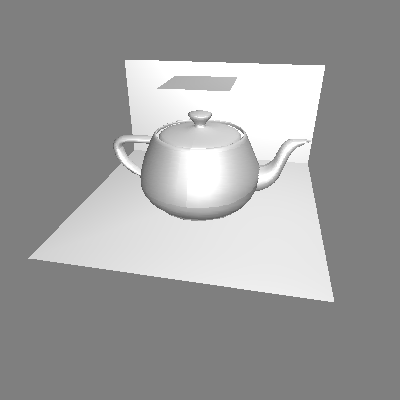Object as viewed from light #2:The Z-buffer algorithm produces shadows not only on z = 0 plane: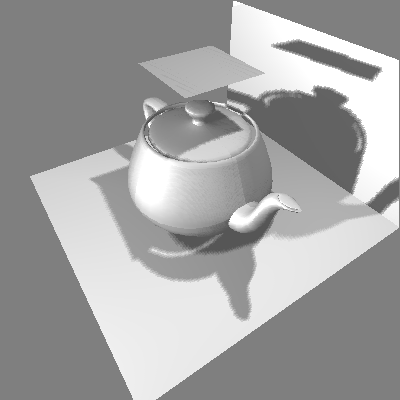## Conclusion

Pros and Cons of Two Algorithms:

A. The Ground Transformation Algorithm

• Requires no extra memory, and easily handles any number of light sources
• However, it only shadows onto ground plane, so it cannot handle objects which shadow other complex objects
• Every polygon is rendered N times, where N is number of light sources

B. The Z-Buffer Algorithm

• Can shadow ANY scene which can be rendered using Z-buffer
• However, it requires a separate memory buffer for each light source
• Again, every polygon is rendered N times, where N is number of light sources, but N-1 views do not need lighting calculations

The Z-buffer algorithm is clearly more versatile, with its ability to add shadows to scenes of arbitrary complexity. Also the precomputed shadow buffers can be used to render views from any eye point as long as the relative positions of the lights and objects are constant between these views. However, if memory resources are limited, the ground transformation algorithm produces pleasing results if only ground shadowing is required.

## References

[WILL78]
Williams, L., "Casting Curved Shadows on Curved Surfaces", Computer Graphics, vol. 12, no. 3, pp270-4, 1978.
[BLIN78]
Blinn, James, "Me and my (fake) shadow", IEEE Computer Graphics and Applications, January 1988.

## Examples

Multiple objects illuminated by two light sources: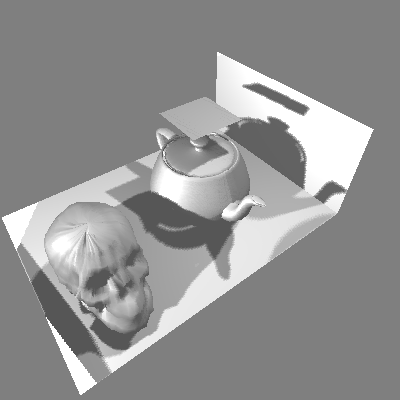Shadowing of texture mapped objects: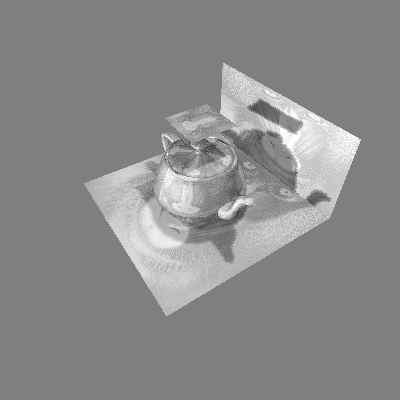Visible surfaces shadowing themselves: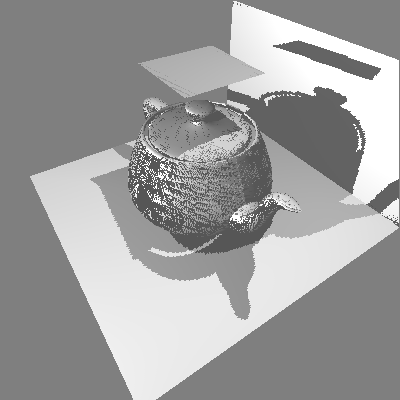Chris Lawson Bentley
chrisb@wpi.edu
Fri Apr 28 14:54:17 EDT 1995